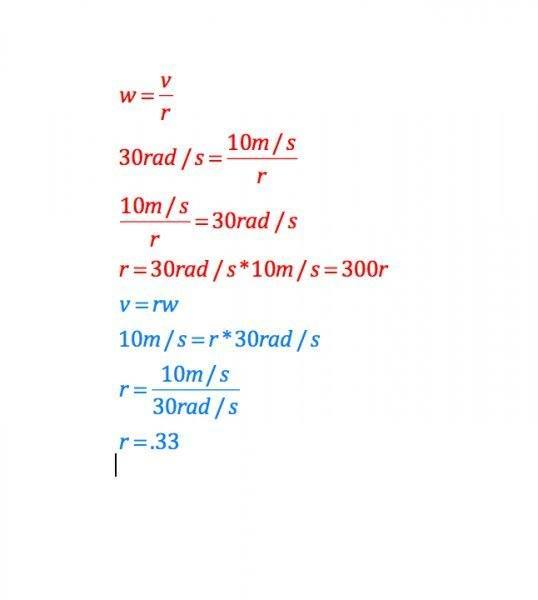# Diameter of bicycle tire

## Homework Statement

A bicycle tire rotates at 30 radians/s. If the bicyclist travels at 10 m/s, what is the diameter of the tire?

## Homework Equations

I found; for angular speed, w=v/r
and for linear speed; v=rw
where v=m/s

So if i am thinking correctly, i already know my angular speed of 30 radians/s
and my linear speed is 10m/s
so now i just need to find the radius or diameter of the tire.

If i try solving for r, i get completely different answers.

## The Attempt at a SolutionBystander
Homework Helper
Gold Member
How did you get the 300 in your first solution? Remember what you're solving for, and that you have to treat both sides of an equation the same way.

How did you get the 300 in your first solution? Remember what you're solving for, and that you have to treat both sides of an equation the same way.
good question.... It's been a long day here at the power plant.

I always tend to get confused when i try to solve for certain things. i was wanting to solve for "r" by multiplying both sides by 10.

so now i am getting; 30rad/s = 10m/s / r
r=10/30
r=.33

so that is just a radius. The diameter would be .66 meters? or 66cm...?

Bystander
Homework Helper
Gold Member
You got that for the second solution, and now for the first solution. That's what I get. And, that should be the diameter.

•keithcuda
You got that for the second solution, and now for the first solution. That's what I get. And, that should be the diameter.
It makes much more sense when I solve for "r" the proper way... :w

Thanks for the help
Cheers
Keith

Bystander